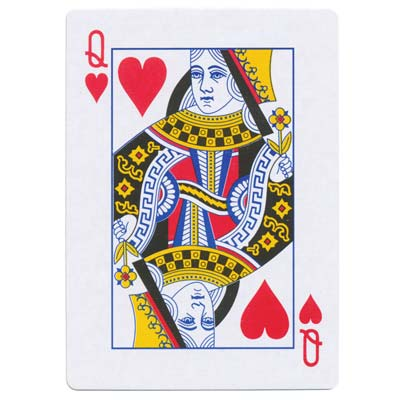## Глосарій

Виберіть одне з ключових слів ліворуч ...

# Transformations and SymmetrySymmetry

Час читання: ~30 min

Symmetry is everywhere around us, and an intuitive concept: different parts of an object look the same in some way. But using transformations, we can give a much more precise, mathematical definition of what symmetry really means:

An object is symmetric if it looks the same, even after applying a certain transformation.We can reflect this butterfly, and it looks the same afterwards. We say that it has reflectional symmetry.We can rotate this flower, and it looks the same afterwards. We say that it has rotational symmetry.

## Reflectional Symmetry

A shape has reflectional symmetry if it looks the same after being reflected. The line of reflection is called the axis of symmetry, and it splits the shape into two halves. Some figures can also have more than one axis of symmetry.

Draw all axes of symmetry in these six images and shapes:

This shape has axes of symmetry.

A square has axes of symmetry.

This shape has axes of symmetry.

Many letters in the alphabet have reflectional symmetry. Select all the ones that do:

A
B
C
D
E
F
G
H
I
J
K
L
M
N
O
P
Q
R
S
T
U
V
W
X
Y
Z

Here are some more shapes. Complete them so that they have reflectional symmetry:

Shapes, letters and images can have reflectional symmetry, but so can entire numbers, words and sentences!

For example “25352” and “ANNA” both read the same from back to front. Numbers or words like this are called Palindromes. Can you think of any other palindromes?

If we ignore spaces and punctuation, the short sentences below also have reflectional symmetry. Can you come up with your own?

Never odd or even.
A for a jar of tuna.
Yo, banana !

But Palindromes are not just fun, they actually have practical importance. A few years ago, scientists discovered that parts of our DNA are palindromic. This makes them more resilient to mutations or damage – because there is a second backup copy of every piece.

## Rotational Symmetry

A shape has rotational symmetry if it looks the same after being rotated (by less than 360°). The center of rotation is usually just the middle of the shape.

The order of symmetry is the number of distinct orientations in which the shape looks the same. You can also think about it as the number of times we can rotate the shape, before we get back to the start. For example, this snowflake has order .

The angle of each rotation is 360°order. In the snowflake, this is 360°6=°.

Find the order and the angle of rotation, for each of these shapes:Order , angle °Order , angle °Order , angle °

Now complete these shapes, so that they have rotational symmetry:

Order 4

Order 2

Order 4

Archie